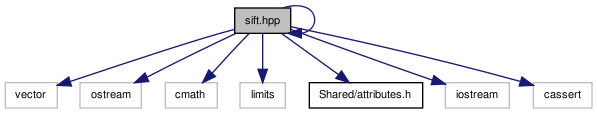# sift.hpp File Reference

`#include <vector>`
`#include <ostream>`
`#include <cmath>`
`#include <limits>`
`#include "Shared/attributes.h"`
`#include "sift.ipp"`
`#include <iostream>`
`#include <cassert>`
Include dependency graph for sift.hpp:This graph shows which files directly or indirectly include this file:Go to the source code of this file.

## Classes

struct  VL::Exception
Generic exception. More...
struct  VL::PgmBuffer
PGM buffer descriptor. More...
class  VL::Sift
SIFT filter. More...
struct  VL::Sift::Keypoint
SIFT keypoint. More...

## Namespaces

namespace  VL

VisionLab namespace.

## Defines

#define VL_FASTFLOAT   float
#define VL_XEAS(x)   #x
#define VL_EXPAND_AND_STRINGIFY(x)   VL_XEAS(x)
#define VL_THROW(x)
Throw generic exception.

## Typedefs

typedef float VL::pixel_t
Pixel data type.
typedef VL_FASTFLOAT VL::float_t
Floating point data type.
typedef float VL::float32_t
32-bit floating data type
typedef double VL::float64_t
64-bit floating data type
typedef int VL::int32_t
32-bit integer data type
typedef int VL::uint32_t
64-bit integer data type
typedef char unsigned VL::uint8_t
8-bit unsigned integer data type

## Functions

Fast math

We provide approximate mathematical functions. These are usually rather faster than the corresponding standard library functions.

float VL::fast_resqrt (float x)
Fast `resqrt`.
double VL::fast_resqrt (double x)
Fast `resqrt`.
float_t VL::fast_expn (VL::float_t x)
Fast @ exp(-x).
float_t VL::fast_abs (VL::float_t x)
Fast `abs(x)`.
float_t VL::fast_mod_2pi (VL::float_t x)
Fast `mod(x,2pi)`.
float_t VL::fast_atan2 (VL::float_t y, VL::float_t x)
Fast `atan2`.
float_t VL::fast_sqrt (VL::float_t x)
Fast `sqrt`.
int32_t VL::fast_floor (VL::float_t x)
Fast (int) floor(x).
PGM input/output

std::ostream & VL::insertPgm (std::ostream &os, pixel_t const *im, int width, int height)
Insert PGM file into stream.
std::istream & VL::extractPgm (std::istream &in, PgmBuffer &buffer)
Extract PGM file from stream.
void VL::createPgmBufferFromArray (int w, int h, pixel_t *d, PgmBuffer &buffer)

## Define Documentation

 #define VL_EXPAND_AND_STRINGIFY ( x ) VL_XEAS(x)

Definition at line 64 of file sift.hpp.

Referenced by siftdriver().

 #define VL_FASTFLOAT   float

Definition at line 60 of file sift.hpp.

Referenced by siftdriver().

 #define VL_THROW ( x )
Value:
```{                                             \
std::ostringstream oss ;                    \
oss << x ;                                  \
throw VL::Exception(oss.str()) ;            \
}
```

Throw generic exception.

The macro executes the stream operations x to obtain an error messages. The message is then wrapped in a generic exception VL::Exception and thrown.

Parameters:
 x sequence of stream operations.

Definition at line 139 of file sift.hpp.

Referenced by siftdriver().

 #define VL_XEAS ( x ) #x

Definition at line 63 of file sift.hpp.

 Tekkotsu v5.1CVS Generated Mon May 9 04:59:02 2016 by Doxygen 1.6.3